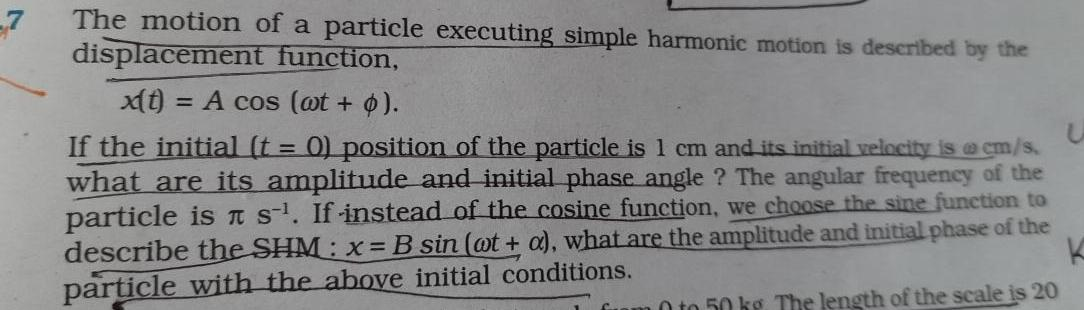Question:

# 7 The motion of a particle executing simple harmonic motion

Last updated: 6/8/20237 The motion of a particle executing simple harmonic motion is described by the displacement function x t A cos wt p If the initial t 0 position of the particle is 1 cm and its initial velocity is cm s what are its amplitude and initial phase angle The angular frequency of the particle is ns If instead of the cosine function we choose the sine function to describe the SHM x B sin at a what are the amplitude and initial phase of the particle with the above initial conditions fuam 0 to 50 kg The length of the scale is 20